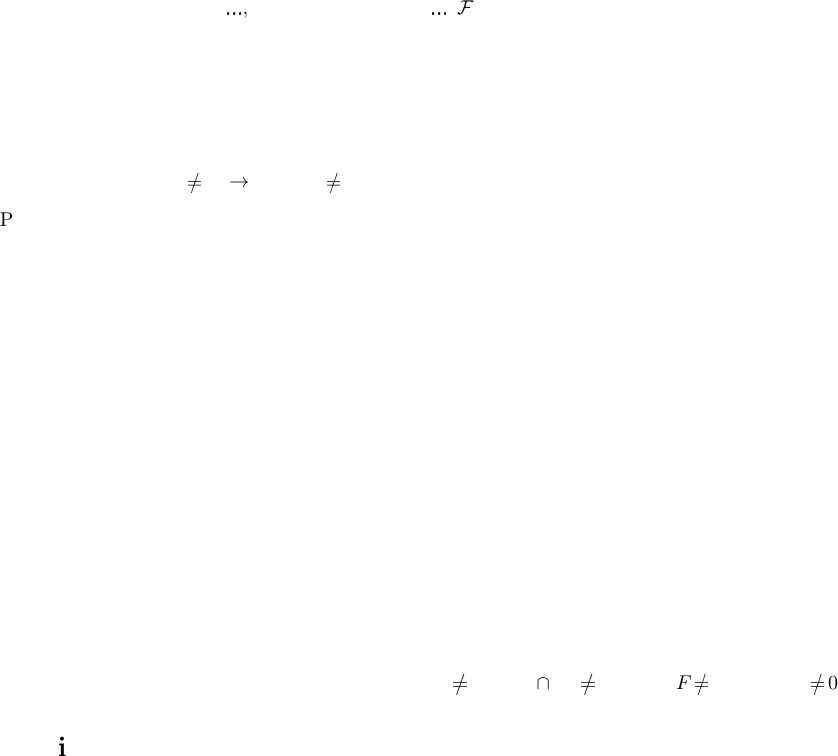Algebraic General Topology. Vol 1: Paperback / E-book || Axiomatic Theory of Formulas: Paperback / E-book

As an Amazon Associate I earn from qualifying purchases.Errata for “Filters on Posets and Generalizations” 
Proposition 7: “Every co-brouwerian lattic e has least element “Every non-empty co-brouwerian
lattice has least element”.
Proof of theorem 17: (a \
b) \
c = {z A | a \
b c z } (a \
b) \
c =
T
{z A | a \
b c z }.
Corollary 17: F is an atomically separable” F is a tomically separable”.
Deﬁnition 38: whenever
S
Z
S exists for S PA “whenever
S
Z
S exists for S PZ”.
Deﬁnition 39: whenever
T
Z
S exists for S PA “whenever
T
Z
S exists for S PZ”.
Theorem 35: “for any F
0
,
, F
m
“for any F
0
,
, F
m
F .
Proof of t he orem 45: “taken into account the theorems 10 and 29” “taken into account the
corollary 10 and theorem 23”.
Theorem 52: a be prime” a is prime”.
Proof of theorem 52: a is prime” a be prime”.
Theorem 54: S F
0 S F
”.
Proof of theorem 56: a
F
b ⋆S a
F
b F and a S b ⋆S a F b F”.
Proof of the ore m 59: “used the t heore ms 29 and 29” “used theorem 29”; “used the theorems 23
and 10 used theorem 23 and corollary 10”.
Theorem 65: “which is an atomistic lattice” “which is a c omplete atomisti c lattice”.
Theorem 68: “for every a, b A “for every a, b F.
Proof of proposition 41: Replace all occurences of A F.
Not yet pubished in IJPAM
Theorem 47: “distributive lattice with least element 0 “distributive lattice with greatest element”
Proof of theorems 12: Messed and .
Proof of theorem 55: card A card T .
Proof of proposition 39: S [S].
Proposition 13: atoms a atoms b a b replace with a b atoms a atoms b.
Proof of theorem 4.53: Should r ead We have L
A
F
0 K
L
Z
F
0 L
Z
F
0 L
A
F
0”.
Bib liography
 Victor Porton. Filters on posets and generalizations. International Journal of Pure and Applied Mathematics,
74(1):55119, 2012.
1